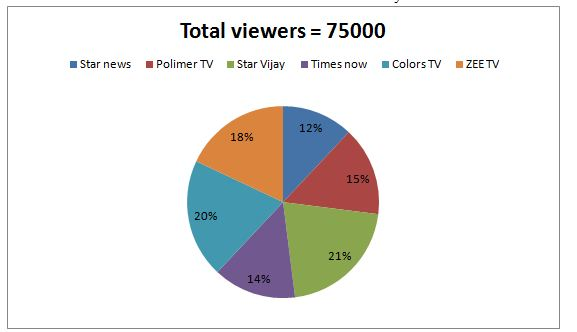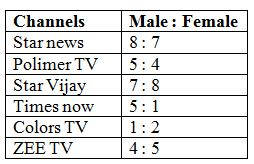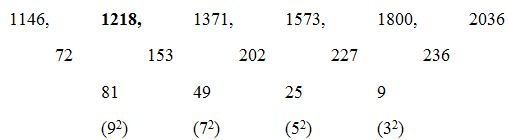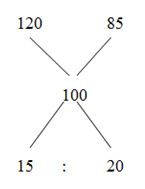# IBPS Clerk 2018 Sectional Revision Test- 2 | Numerical Ability

Dear Readers, Take Free Numerical Ability Sectional Test of 35 Questions as like in the real exam to analyze your preparation level. Our Sectional Test Questions are taken as per the latest exam pattern and so it will be really useful for you to crack the prelims exam lucratively. Students who are weak in Numerical Ability should utilize this chance constructively to accomplish a successful profession in Banking Field.

[WpProQuiz 4481]

Directions (Q. 1 – 5): Each question contains a statement followed by Quantity I and Quantity II. Read the contents clearly and answer your questions accordingly.

1) Quantity I: The area of rectangular garden is 2080 Sq m. The length of the rectangular garden is 30 % more than the breadth. Find the perimeter of the garden?

Quantity II: The area of a square park is 2304 Sq m. Find the perimeter of the park?

a) Quantity I > Quantity II

b) Quantity I ≥ Quantity II

c) Quantity II > Quantity I

d) Quantity II ≥ Quantity I

e) Quantity I = Quantity II or Relation cannot be established

2) Quantity I: The shopkeeper sold an article at 15 % discount on marked price and he gains 25 %. If the marked price of the article is Rs. 600, then the cost price is?

Quantity II: The shopkeeper marks the price of the book Rs. 550 and his profit % is 20 %. Find the cost price of the book, if he allows a discount of 16 %?

a) Quantity I > Quantity II

b) Quantity I ≥ Quantity II

c) Quantity II > Quantity I

d) Quantity II ≥ Quantity I

e) Quantity I = Quantity II or Relation cannot be established

3) Quantity I: 240 m long train can crosses a pole in 8 sec. The speed of the train is,

Quantity II: 200 m long train can crosses a platform of length 160 m in 24 sec. The speed of the train is,

a) Quantity I > Quantity II

b) Quantity I ≥ Quantity II

c) Quantity II > Quantity I

d) Quantity II ≥ Quantity I

e) Quantity I = Quantity II or Relation cannot be established

4) A bag contains, 4 pink, 7 yellow and 5 black balls.

Quantity I: If 3 balls are drawn randomly, then find the probability of getting at least one yellow ball?

Quantity II: If 2 balls are drawn randomly, then find the probability of getting both the balls are either pink or black?

a) Quantity I > Quantity II

b) Quantity I ≥ Quantity II

c) Quantity II > Quantity I

d) Quantity II ≥ Quantity I

e) Quantity I = Quantity II or Relation cannot be established

5) Quantity I: A and B started a business by Rs. 5400 and Rs. 7200 respectively. A invested only 6 months and they divided their shares after a year. The ratio of profit of A and B is 3 : 8. Then how many months B invested the money?

Quantity II: P and Q invested in the ratio of 7 : 10. P invested the money for 10 months. The ratio of profit of P and Q is 7 : 12. Then, how many months Q invested the money?

a) Quantity I > Quantity II

b) Quantity I ≥ Quantity II

c) Quantity II > Quantity I

d) Quantity II ≥ Quantity I

e) Quantity I = Quantity II or Relation cannot be established

Directions (Q. 6 – 10) Study the following information carefully and answer the given questions:

The following pie chart shows the percentage distribution of total number of television viewers of different channels in a certain city.The following table shows the ratio of male and female television viewers.6) Find the difference between the total number of male television viewers of Star news to that of total number of female television viewers of Star Vijay?

a) 2400

b) 2800

c) 3600

d) 3200

e) None of these

7) Find the ratio between the total number of television viewers of Polimer TV to that of total number of female television viewers of Colors TV?

a) 9 : 8

b) 5 : 3

c) 11 : 7

d) 23 : 15

e) None of these

8) Total number of television viewers of Times Now is what percentage of total number of male television viewers of ZEE TV?

a) 215 %

b) 160 %

c) 140 %

d) 175 %

e) None of these

9) Find the average number of male television viewers of Polimer TV, Star Vijay and Colors TV together?

a) 5800

b) 6200

c) 6350

d) 6700

e) None of these

10) Total number of female television viewers of Star news is what percentage more/less than the total number of male television viewers of ZEE TV?

a) 10 % more

b) 20 % less

c) 30 % less

d) 25 % more

e) None of these

Directions (Q. 11 – 15): What value should come in place of question mark (?) in the following questions?

11) √16129 + 3944312 + 66 % of 1800 = 579 + ?

a) 725

b) 636

c) 978

d) 894

e) None of these

12) (4/5) of (95/29) of 4350 = 36 % of 2700 + (4/7) of (?)

a) 18249

b) 19456

c) 21324

d) 15420

e) None of these

13) 522 ÷ 14 × 7 + 452 = 5 × ? – 35 % of 3100

a) 814.6

b) 728

c) 892.4

d) 654

e) None of these

14) 3224 ÷ (31 % of 1300) = 49 × 9 2/7 of (? ÷ 130)

a) 17/9

b) 16/7

c) 24/5

d) 33/5

e) None of these

15) (13 × 11 + 48 × 7 + 8 × 9 + 47 × 2) ÷ (122 – √1156 + 19) = ?

a) 12

b) 24

c) 9

d) 5

e) None of these

Directions (Q. 16 – 20) Find the wrong term in the following number series?

16) 1146, 1217, 1371, 1573, 1800, 2036

a) 1371

b) 1573

c) 1217

d) 2036

e) 1800

17) 225, 223, 111, 36, 8

a) 223

b) 111

c) 36

d) 225

e) 8

18) 4, 26, 178, 1238, 8672

a) 26

b) 4

c) 1238

d) 178

e) 8672

19) 21, 10, 11, 16.5, 32, 77.5, 235.5

a) 10

b) 38

c) 97.5

d) 295.5

e) 5

20) 37, 40, 85, 267, 1080, 5415

a) 37

b) 267

c) 1080

d) 85

e) 5415

21) Mahesh can do a work in 24 days. He works alone for 6 days and Naveen alone finishes the remaining work in 12 days. Both of them together can complete the work in?

a) 7 2/7 days

b) 15 ¼ days

c) 12 2/3 days

d) 9 3/5 days

e) None of these

22) A, B and C started a business by investing Rs. 40500, Rs. 45000 and Rs. 60000 respectively. After 6 months C withdrew Rs. 15000 while A invested Rs. 4500 more. If the total profit at the end of the year is Rs. 149600, then the share of C will exceed that of A by?

a) Rs. 19000

b) Rs. 11500

c) Rs. 13000

d) Rs. 10400

e) None of these

23) The simple interest accrued on an amount of Rs. 17,000 at the end of 4 years is Rs. 6800. What would be the compound interest accrued on the same amount at the same rate in the same period?

a) Rs. 7889.70

b) Rs. 8324.20

c) Rs. 6990.50

d) Rs. 7129.30

e) Rs. 7558.60

24) Smallest angle of a triangle is equal to two-third of the smallest angle of a quadrilateral. The ratio between the angles of the quadrilateral is 3 : 4 : 5 : 6. Largest angle of the triangle is twice its smallest angle. What is the sum of second largest angle of the triangle and largest angle of the quadrilateral?

a) 160°

b) 180°

c) 190°

d) 170°

e) 150°

25) If the numerator of a fraction is increased by 150% and the denominator of the fraction is increased by 350%, the resultant fraction becomes 25/51. Then find the original fraction?

a) 13/15

b) 11/17

c) 11/15

d) 15/17

e) None of these

26) A boat can cover 15 km downstream in 45 minutes. If the speed of the current is ¼ of the boat in still water, then how much distance can the boat cover upstream in 66 minutes?

a) 13.2 km

b) 16 km

c) 18 km

d) 10.8 km

e) None of these

27) A man purchases 2 watches for Rs. 665. He sells one of them at 20% profit and another at 15% loss. Thus, he neither gains nor loses. Find the selling price?

a) Rs. 342, Rs. 323

b) Rs. 285, Rs. 380

c) Rs. 410, Rs. 353

d) Rs. 267, Rs. 323

e) None of these

28) A bag contains 4 vivo mobiles, 6 Lenovo mobiles and 5 oppo mobiles. 3 mobiles are drawn at random. What is the probability of choosing at least one is oppo mobile?

a) 24/91

b) 43/91

c) 57/91

d) 67/91

e) None of these

29) Ravi has 35 % savings with him from his monthly salary. If the expenditure of clothing is 20 % of overall expenditure and his expenditure except clothing is Rs. 10400. Find his savings?

a) Rs. 6500

b) Rs. 5850

c) Rs. 7000

d) Rs. 7150

e) None of these

30) Priya is 7 years older than Revathi. The ratio of the present ages of Priya to that of Mini is 3 : 4. Revathi is 15 years younger than Mini. What is Revathi’s present age?

a) 23 years

b) 20 years

c) 25 years

d) 17 years

e) None of these

given. You have to solve both the equations and give answer as,

a) If x > y

b) If x ≥ y

c) If x < y

d) If x ≤ y

e) If x = y or the relation cannot be established

31)

I) x2 – 12x + 36 = 0

II) 5y2 + 4y – 12 = 0

32)

I) 2x2 + 12x + 16 = 0

II) 3y2 -7y – 6 = 0

33)

I) 7x + 2y = -13

II) 5x – 4y = 7

34)

I) x2 + 2x – 15 = 0

II) y2 + y – 56 = 0

35)

I) ∜625 x + √5625 = 0

II) (243)1/5 y + (1728)1/3 = 0

Directions (Q. 1 – 5):

Quantity I:

The area of rectangular garden = 2080 Sq m

l/b = 13/10 = > l : b = 13 : 10

13x*10x = 2080

130x2 = 2080

X2 = (2080/130) = 16

X = 4

Length = 52 m, Breadth = 40 m

Perimeter of the garden = 2*(l + b) = 2*(52 + 40) =2*92 = 184 m

Quantity II:

The area of a square park = 2304 Sq m

Area (a2) = 2304

Side (a) = 48

Perimeter of the park = 4a = 4*48 = 192 m

Quantity II > Quantity I

Quantity I:

Selling price of the article = 600*(85/100) = Rs. 510

Cost price of the article

= > CP*(125/100) = 510

= > CP = 510*(100/125)

= > CP = Rs. 408

Quantity II:

Selling price of the book = 550*(84/100) = Rs. 462

Cost price of the book = 462*(100/120) = Rs. 385

Quantity I > Quantity II

Quantity I:

Speed = Distance/Time

= > 240/8

= > 30 m/sec

The speed of the train = 30 m/sec

Quantity II:

Distance = Length of train + Platform length = 200 + 160 = 360 m

Speed = 360/24 = 15 m/sec

Quantity I > Quantity II

Total no of balls = 4 + 7 + 5 = 16 balls

Quantity I:

n(S) = 16C3 = (16*15*14)/(1*2*3)

The probability of getting at least one yellow ball = 1- P (None is yellow ball)

P (None is yellow ball)

n(E) = 9C3 = (9*8*7)/(1*2*3)

P(E) = n(E)/n(S) = 9C3 /16C3

= > [(9*8*7)/(1*2*3)] / [(16*15*14)/(1*2*3)]

= > 3/20

Required probability = 1 – (3/20) = 17/20

Quantity II:

n(S) = 16C2 = (16*15)/(1*2)

n(E) = Probability that both the balls are either pink or black

n(E) = 4C2 or 5C2

P(E) = n(E)/n(S)

= > [4C2 or 5C2] / 16C2

= > [6 + 10] / [(16*15)/(1*2)]

= > (16*2)/(16*15) = 2/15

Quantity I > Quantity II

Quantity I:

According to the question,

(5400*6)/(7200*x) = (3/8)

32400/7200x = 3/8

32400*8 = 7200x*3

X = (32400*8)/(7200*3)

X = 12 months

Quantity II:

According to the question,

(7*10)/(10x) = (7/12)

X = 12 months

Quantity I = Quantity II

Directions (Q. 6 – 10):

The total number of male television viewers of Star news

= > 75000*(12/100)*(8/15) = 4800

The total number of female television viewers of Star Vijay

= > 75000*(21/100)*(8/15) = 8400

Required difference = 8400 – 4800 = 3600

The total number of television viewers of Polimer TV

= > 75000*(15/100) = 11250

The total number of female television viewers of Colors TV

= > 75000*(20/100)*(2/3) = 10000

Required ratio = 11250 : 10000 = 9 : 8

Total number of television viewers of Times Now

= > 75000*(14/100) = 10500

Total number of male television viewers of ZEE TV

= > 75000*(18/100)*(4/9) = 6000

Required % = (10500/6000)*100 = 175 %

The total number of male television viewers of Polimer TV, Star Vijay and Colors TV together

= > 75000*(15/100)*(5/9) + 75000*(21/100)*(7/15) + 75000*(20/100)*(1/3)

= > 6250 + 7350 + 5000 = 18600

Required average = 18600/3 = 6200

Total number of female television viewers of Star news

= > 75000*(12/100)*(7/15) = 4200

Total number of male television viewers of ZEE TV

= > 75000*(18/100)*(4/9) = 6000

Required % = [(6000 – 4200)/6000]*100 = 30 % less

Directions (Q. 11 – 15):

127 + 158 + (66/100)*1800 – 579 = x

X = 127 + 158 + 1188 – 579

X = 894

(4/5)*(95/29)*4350 = (36/100)*2700 + (4/7)*x

11400 – 972 = (4x/7)

10428*(7/4) = x

X = 18249

(52*52*7)/14 + 452 = 5x – (35/100)*3100

1352 + 2025 = 5x – 1085

1352 + 2025 + 1085 = 5x

X = 4462/5

X = 892.4

3224 ÷ [(31/100)*1300] = 49*(65/7)*(x/130)

3224/403 = (7x/2)

8*(2/7) = x

X = 16/7

(13 × 11 + 48 × 7 + 8 × 9 + 47 × 2) ÷ (122 – √1156 + 19) = x

X = (143 + 336 + 72 + 94) / (144 – 34 + 19)

X = 645/129 = 5

Directions (Q. 16 – 20):

The correct series is,The difference of difference is, 92, 72, 52, 32,..

The wrong term is, 1217

The correct series is,

225, 224, 111, 36, 8

The pattern is,

= > (225-1)/1 = 224, (224-2)/2 = 111, (111-3)/3 = 36, (36- 4)/4 = 8

The wrong term is, 223

The correct series is,

4, 26, 178, 1240, 8672

The pattern is, *7 – 2, *7 – 4, *7 – 6, *7 -8,..

The wrong term is, 1238

The correct series is,

21, 10, 11, 15, 32, 77.5, 235.5

The pattern is, *0.5 – 0.5, *1 + 1, *1.5 -1.5, *2 + 2, *2.5 – 2.5, *3 + 3,..

The wrong term is, 16.5

The correct series is,

37, 40, 86, 267, 1080, 5415

The pattern is, *1 + 3, *2 + 6, *3 + 9, *4 + 12, *5 + 15,..

The wrong term is, 85

Mahesh one day work = 1/24

6 days work = 6/24 = 1/4

Remaining work = 1 – (1/4) = 3/4

Naveen alone finishes the remaining work in 12 days.

= > (3/4) * Naveen’s whole work = 12

= > Naveen’s whole work = 16 days

Mahesh and Naveen’s one day work,

= > (1/24) + (1/16)

= > 5/48

Both Mahesh and Naveen together complete the work in,

= > 48/5 days = 9 3/5 days

The share of A, B and C

= > (40500*6 + 45000*6) : (45000*12) : (60000*6 + 45000*6)

= > 513000 : 540000 : 630000

= > 57 : 60 : 70

187’s = 149600

1’s = 800

The difference between the share of A to that of C

= > 13’s = 13*800 = Rs. 10400

S.I = PNR/100

6800 = (17000*4*R)/100

R = (6800*100)/(17000*4) = 10 %

C.I = P*(1 + (R/100))n – 1)

= > 17000*(1 + (10/100))4 – 1)

= > 17000*(110/100)4 – 1)

= > 17000*[(14641/10000) – 1]

= > 17000*(4641/10000)

= > Rs. 7889.70

The ratio between the angles of the quadrilateral = 3 : 4 : 5 : 6

3x + 4x + 5x + 6x = 360

=> 18x = 360

=> x = 20

Smallest angle of triangle = (2/3)*60 = 40

Largest angle of the triangle = 2*40 = 80

Second largest angle of the triangle = 180 – 40 – 80 = 60

Required sum = 60 + 120 = 180°

The original fraction is,

= > [x*(250/100)]/[y*(450/100)] = (25/51)

= > x/y = (25/51)*(450/250) = 15/17

The speed of downstream= D/T = 15/(45/60) = 20 km/hr

The speed of the current = ¼ of the boat in still water

Speed of boat : Speed of Current = 4 : 1 = (4x: x)

Speed of downstream = Speed of boat + Speed of Current = 5x

20 = 5x

X= 4

Speed of boat = 16 km/hr, Speed of Current = 4 km/hr

Speed of upstream= Speed of boat – Speed of Current = 3x

Speed of upstream = 12 km/hr

Time = 66 min= (66/60) hr

Distance= S*T = 12*(66/60) = 13.2 km

CP1 + CP2 = 665=> 3 : 4

7’s =665 = > 1’s = 95

CP1 = 95*3 = 285

CP2 = 95*4 = 380

SP1 = 285*(120/100) = Rs. 342

SP2 = 380*(85/100) = Rs. 323

Total probability = 15C3

Required probability = 1- none is oppo mobile

The probability of choosing none is oppo mobile,

= > 10C3/15C3 = (10*9*8)/(15*14*13)

= > 24/91

Probability of choosing at least one is oppo mobile,

= > 1 – (24/91) = 67/91

Savings = 35 %

Expenditure = 65 %

According to the question,

= > (80/100)*expenditure = 10400

Expenditure = 13000

(65/100)*monthly salary = 13000

Monthly salary = 13000*(100/65) = Rs. 20000

Savings = 35 % of monthly salary = (35/100)*20000

= > Rs. 7000

Let the present age of Revathi be x years,

Then, Priya’s age= (x+7) years

Revathi is 12 years younger than Mini

Mini’s age= (x+15) years

So, (x+7)/(x+15) = ¾

x= 17

Revathi’s present age = 17 years

Directions (Q. 31 – 35):

I) x2 – 12x + 36 = 0

(x – 6)(x – 6) = 0

X = 6, 6

II) 5y2 + 4y – 12 = 0

5y2 + 10y – 6y – 12 = 0

5y (y + 2) – 6 (y + 2) = 0

(5y – 6) (y + 2)  = 0

Y = 6/5, – 2 = 1.2, -2

x > y

I) 2x2 + 12x + 16 = 0

2x2 + 4x + 8x + 16 = 0

2x(x + 2) + 8(x + 2) = 0

(2x + 8)(x + 2) = 0

X = -4, -2

II) 3y2 -7y – 6 = 0

3y2 -9y + 2y – 6 = 0

3y(y – 3) + 2(y – 3) = 0

(3y + 2) (y – 3) = 0

Y = – 2/3, 3 = -0.667, 3

x < y

7x + 2y = -13–> (1)

5x – 4y = 7–> (2)

By solving the equation (1) and (2), we get,

X = – 1, y = -3

X > y

I) x2 + 2x – 15 = 0

(x + 5) (x – 3) = 0

X = -5, 3

II) y2 + y – 56 = 0

(y + 8) (y – 7) = 0

Y = -8, 7

Can’t be determined

I) ∜625 x + √5625 = 0

5x = – 75

X = -15

II) (243)1/5 y + (1728)1/3 = 0

(35)1/5 y = – (123)1/3

3y = -12

Y = -4

X < y